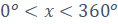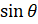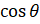### TRIGONOMETRY (GRAPHS OF TRIGONOMETRICAL FUNCTIONS)

Mathematics SSS 3 First Term

WEEK 6

TRIGONOMETRY (GRAPHS OF TRIGONOMETRICAL FUNCTIONS)

Performance Objectives

Student should be able to:

Construct table of values for, plot the graphs of the table of values and interpreting and reading out given values.

Content

Sine and Cosine Graphs

The diagram above shows how the sine and cosine graphs looks like but before we proceed to solving / plotting our graphs, it is important we note a few key points

1. All values of sinθand cosθlie between +1 and – 1.
2. The sine and cosine curves have the same wave shape but they start from different points.
3. View More